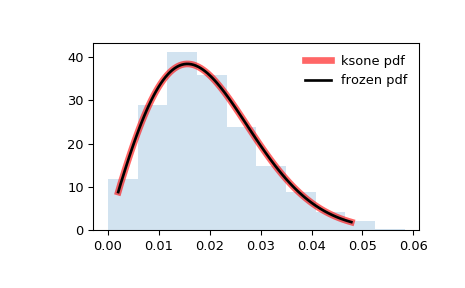# scipy.stats.ksone¶

scipy.stats.ksone = <scipy.stats._continuous_distns.ksone_gen object>[source]

General Kolmogorov-Smirnov one-sided test.

This is the distribution of the one-sided Kolmogorov-Smirnov (KS) statistics $$D_n^+$$ and $$D_n^-$$ for a finite sample size n (the shape parameter).

As an instance of the rv_continuous class, ksone object inherits from it a collection of generic methods (see below for the full list), and completes them with details specific for this particular distribution.

Notes

$$D_n^+$$ and $$D_n^-$$ are given by

$\begin{split}D_n^+ &= \text{sup}_x (F_n(x) - F(x)),\\ D_n^- &= \text{sup}_x (F(x) - F_n(x)),\\\end{split}$

where $$F$$ is a CDF and $$F_n$$ is an empirical CDF. ksone describes the distribution under the null hypothesis of the KS test that the empirical CDF corresponds to $$n$$ i.i.d. random variates with CDF $$F$$.

The probability density above is defined in the “standardized” form. To shift and/or scale the distribution use the loc and scale parameters. Specifically, ksone.pdf(x, n, loc, scale) is identically equivalent to ksone.pdf(y, n) / scale with y = (x - loc) / scale.

References

1

Birnbaum, Z. W. and Tingey, F.H. “One-sided confidence contours for probability distribution functions”, The Annals of Mathematical Statistics, 22(4), pp 592-596 (1951).

Examples

>>> from scipy.stats import ksone
>>> import matplotlib.pyplot as plt
>>> fig, ax = plt.subplots(1, 1)


Calculate a few first moments:

>>> n = 1e+03
>>> mean, var, skew, kurt = ksone.stats(n, moments='mvsk')


Display the probability density function (pdf):

>>> x = np.linspace(ksone.ppf(0.01, n),
...                 ksone.ppf(0.99, n), 100)
>>> ax.plot(x, ksone.pdf(x, n),
...        'r-', lw=5, alpha=0.6, label='ksone pdf')


Alternatively, the distribution object can be called (as a function) to fix the shape, location and scale parameters. This returns a “frozen” RV object holding the given parameters fixed.

Freeze the distribution and display the frozen pdf:

>>> rv = ksone(n)
>>> ax.plot(x, rv.pdf(x), 'k-', lw=2, label='frozen pdf')


Check accuracy of cdf and ppf:

>>> vals = ksone.ppf([0.001, 0.5, 0.999], n)
>>> np.allclose([0.001, 0.5, 0.999], ksone.cdf(vals, n))
True


Generate random numbers:

>>> r = ksone.rvs(n, size=1000)


And compare the histogram:

>>> ax.hist(r, density=True, histtype='stepfilled', alpha=0.2)
>>> ax.legend(loc='best', frameon=False)
>>> plt.show()Methods

 rvs(n, loc=0, scale=1, size=1, random_state=None) Random variates. pdf(x, n, loc=0, scale=1) Probability density function. logpdf(x, n, loc=0, scale=1) Log of the probability density function. cdf(x, n, loc=0, scale=1) Cumulative distribution function. logcdf(x, n, loc=0, scale=1) Log of the cumulative distribution function. sf(x, n, loc=0, scale=1) Survival function (also defined as 1 - cdf, but sf is sometimes more accurate). logsf(x, n, loc=0, scale=1) Log of the survival function. ppf(q, n, loc=0, scale=1) Percent point function (inverse of cdf — percentiles). isf(q, n, loc=0, scale=1) Inverse survival function (inverse of sf). moment(n, n, loc=0, scale=1) Non-central moment of order n stats(n, loc=0, scale=1, moments=’mv’) Mean(‘m’), variance(‘v’), skew(‘s’), and/or kurtosis(‘k’). entropy(n, loc=0, scale=1) (Differential) entropy of the RV. fit(data, n, loc=0, scale=1) Parameter estimates for generic data. expect(func, args=(n,), loc=0, scale=1, lb=None, ub=None, conditional=False, **kwds) Expected value of a function (of one argument) with respect to the distribution. median(n, loc=0, scale=1) Median of the distribution. mean(n, loc=0, scale=1) Mean of the distribution. var(n, loc=0, scale=1) Variance of the distribution. std(n, loc=0, scale=1) Standard deviation of the distribution. interval(alpha, n, loc=0, scale=1) Endpoints of the range that contains alpha percent of the distribution

#### Previous topic

scipy.stats.kappa3

#### Next topic

scipy.stats.kstwobign